# Angle trisection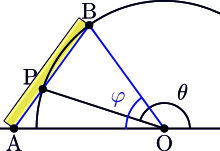Angles may be trisected via a Neusis construction, but this uses tools outside the Greek framework of an unmarked straightedge and a compass.

Angle trisection is a classical problem of compass and straightedge constructions of ancient Greek mathematics. It concerns construction of an angle equal to one third of a given arbitrary angle, using only two tools: an unmarked straightedge, and a compass.

The problem as stated is generally impossible to solve, as shown by Pierre Wantzel in 1837. However, although there is no way to trisect an angle in general with just a compass and a straightedge, some special angles can be trisected. For example, it is relatively straightforward to trisect a right angle (that is, to construct an angle of measure 30 degrees).

It is possible to trisect an arbitrary angle by using tools other than straightedge and compass. For example, neusis construction, also known to ancient Greeks, involves simultaneous sliding and rotation of a marked straightedge, which cannot be achieved with the original tools. Other techniques were developed by mathematicians over the centuries.

Because it is defined in simple terms, but complex to prove unsolvable, the problem of angle trisection is a frequent subject of pseudomathematical attempts at solution by naive enthusiasts. These "solutions" often involve mistaken interpretations of the rules, or are simply incorrect.

## Background and problem statement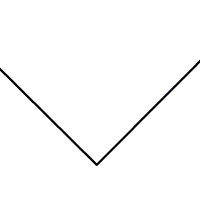Bisection of arbitrary angles has long been solved.

Using only an unmarked straightedge and a compass, Greek mathematicians found means to divide a line into an arbitrary set of equal segments, to draw parallel lines, to bisect angles, to construct many polygons, and to construct squares of equal or twice the area of a given polygon.

Three problems proved elusive, specifically, trisecting the angle, doubling the cube, and squaring the circle. The problem of angle trisection reads:

Construct an angle equal to one-third of a given arbitrary angle (or divide it into three equal angles), using only two tools:

1. an unmarked straightedge, and
2. a compass.

## Proof of impossibility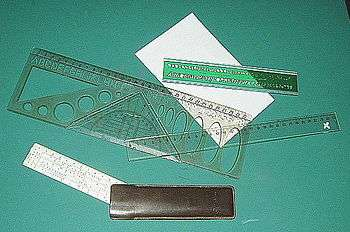Rulers. The displayed ones are marked — an ideal straightedge is un-marked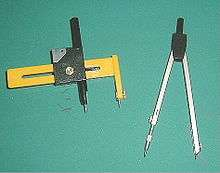Pierre Wantzel published a proof of the impossibility of classically trisecting an arbitrary angle in 1837. Wantzel's proof, restated in modern terminology, uses the abstract algebra of field extensions, a topic now typically combined with Galois theory. However Wantzel published these results earlier than Galois (whose work was published in 1846) and did not use the connection between field extensions and groups that is the subject of Galois theory itself.

The problem of constructing an angle of a given measure θ is equivalent to constructing two segments such that the ratio of their length is cos θ. From a solution to one of these two problems, one may pass to a solution of the other by a compass and straightedge construction. The triple-angle formula gives an expression relating the cosines of the original angle and its trisection: cos θ = 4cos3(θ/3)  3cos(θ/3). It follows that, given a segment that is defined to have unit length, the problem of angle trisection is equivalent to constructing a segment whose length is the root of a cubic polynomial. This equivalence reduces the original geometric problem to a purely algebraic problem.

Every rational number is constructible. Every irrational number which is constructible in a single step from some given numbers is a root of a polynomial of degree 2 with coefficients in the field generated by these numbers. Therefore, any number which is constructible by a sequence of steps is a root of a minimal polynomial whose degree is a power of two. Note also that π/3 radians (60 degrees, written 60°) is constructible. The argument below shows that it is impossible to construct a 20° angle. This implies that a 60° angle cannot be trisected, and thus that an arbitrary angle cannot be trisected.

Denote the set of rational numbers by Q. If 60° could be trisected, the degree of a minimal polynomial of cos(20°) over Q would be a power of two. Now let y = cos(20°). Note that cos(60°) = cos(π/3) = 1/2. Then by the triple-angle formula, cos(π/3) = 1/2 = 4y3 − 3y and so 4y3 − 3y − 1/2 = 0. Thus 8y3 − 6y − 1 = 0, or equivalently (2y)3 − 3(2y) − 1 = 0. Now substitute x = 2y, so that x3 − 3x − 1 = 0. Let p(x) = x3 − 3x − 1.

The minimal polynomial for x (hence cos(20°)) is a factor of p(x). Because p(x) has degree 3, if it is reducible over by Q then it has a rational root. By the rational root theorem, this root must be 1 or −1, but both are clearly not roots. Therefore, p(x) is irreducible over by Q, and the minimal polynomial for cos(20°) is of degree 3.

So an angle of 60° = π/3 radians cannot be trisected.

## Angles which can be trisected

However, some angles can be trisected. For example, for any constructible angle θ, the angle 3θ can be trivially trisected by ignoring the given angle and directly constructing an angle of measure θ. There are angles which are not constructible, but are trisectible despite the one-third angle itself being non-constructible. For example, 3π7 is such an angle: five copies of 3π7 combine to make an angle of measure 15π7, which is a full circle plus the desired π7.

For a positive integer N, an angle of measure 2πN is trisectible if and only if 3 does not divide N. In contrast, 2πN is constructible if and only if N is a power of 2 or the product of a power of 2 with the product of one or more distinct Fermat primes.

### Algebraic characterization

Again, denote the set of rational numbers by Q:

Theorem: The angle θ may be trisected if and only if q(t) = 4t3 − 3t − cos(θ) is reducible over the field extension Q(cos(θ)).

The proof is a relatively straightforward generalization of the proof given above that a 60° angle is not trisectible.

## Other methods

The general problem of angle trisection is solvable by using additional tools, and thus going outside of the original Greek framework of compass and straightedge.

Many incorrect methods of trisecting the general angle have been proposed. Some of these methods provide reasonable approximations; others (some of which are mentioned below) involve tools not permitted in the classical problem. The mathematician Underwood Dudley has detailed some of these failed attempts in his book The Trisectors.

### Approximation by successive bisections

Trisection can be approximated by repetition of the compass and straightedge method for bisecting an angle. The geometric series 1/3 = 1/4 + 1/16 + 1/64 + 1/256 + ⋯ or 1/3 = 1/2-1/4+1/8-1/16+... can be used as a basis for the bisections. An approximation to any degree of accuracy can be obtained in a finite number of steps.

### Using origami

Trisection, like many constructions impossible by ruler and compass, can easily be accomplished by the more powerful operations of paper folding, or origami. Huzita's axioms (types of folding operations) can construct cubic extensions (cube roots) of given lengths, whereas ruler-and-compass can construct only quadratic extensions (square roots).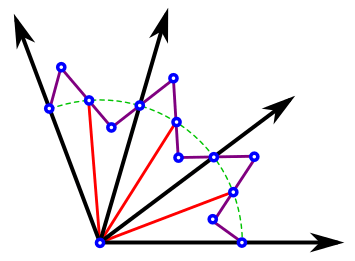There are a number of simple linkages which can be used to make an instrument to trisect angles including Kempe's Trisector and Sylvester's Link Fan or Isoklinostat.

### With an auxiliary curve

There are certain curves called trisectrices which, if drawn on the plane using other methods, can be used to trisect arbitrary angles.

### With a marked ruler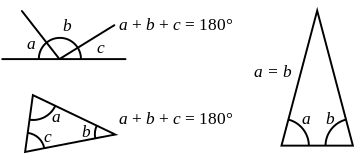Basic triangle's angle properties

Another means to trisect an arbitrary angle by a "small" step outside the Greek framework is via a ruler with two marks a set distance apart. The next construction is originally due to Archimedes, called a Neusis construction, i.e., that uses tools other than an un-marked straightedge. The diagrams we use show this construction for an acute angle, but it indeed works for any angle up to 180 degrees.

This requires three facts from geometry (at right):

1. Any full set of angles on a straight line add to 180°,
2. The sum of angles of any triangle is 180°, and,
3. Any two equal sides of an isosceles triangle will meet the third in the same angle.Trisection of the angle using marked ruler

Let l be the horizontal line in the diagram on the right. Angle a (left of point B) is the subject of trisection. First, a point A is drawn at an angle's ray, one unit apart from B. A circle of radius AB is drawn. Then, the markedness of the ruler comes into play: one mark of the ruler is placed at A and the other at B. While keeping the ruler (but not the mark) touching A, the ruler is slid and rotated until one mark is on the circle and the other is on the line l. The mark on the circle is labeled C and the mark on the line is labeled D. This ensures that CD = AB. A radius BC is drawn to make it obvious that line segments AB, BC, and CD all have equal length. Now, triangles ABC and BCD are isosceles, thus (by Fact 3 above) each has two equal angles.

Hypothesis: Given AD is a straight line, and AB, BC, and CD all have equal length,

Conclusion: angle b = a/3.

1. From Fact 1) above,°.
2. Looking at triangle BCD, from Fact 2)°.
3. From the last two equations,.
4. From Fact 2),°, thus°, so from last,°.
5. From Fact 1) above,°, thus°°.

Clearing, a − 3b = 0, or a = 3b, and the theorem is proved.

Again, this construction stepped outside the framework of allowed constructions by using a marked straightedge.

### With a string

Thomas Hutcheson published an article in the Mathematics Teacher that used a string instead of a compass and straight edge. A string can be used as either a straight edge (by stretching it) or a compass (by fixing one point and identifying another), but can also wrap around a cylinder, the key to Hutcheson's solution.

Hutcheson constructed a cylinder from the angle to be trisected by drawing an arc across the angle, completing it as a circle, and constructing from that circle a cylinder on which a, say, equilateral triangle was inscribed (a 360-degree angle divided in three). This was then "mapped" onto the angle to be trisected, with a simple proof of similar triangles.

### With a "tomahawk"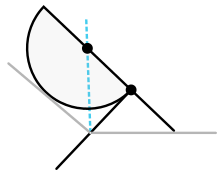A tomahawk trisecting an angle. The handle forms one trisector and the blue line shown forms the other.

A "tomahawk" is a geometric shape consisting of a semicircle and two orthogonal line segments, such that the length of the shorter segment is equal to the circle radius. Trisection is executed by leaning the end of the tomahawk's shorter segment on one ray, the circle's edge on the other, so that the "handle" (longer segment) crosses the angle's vertex; the trisection line runs between the vertex and the center of the semicircle.

Note that while a tomahawk is constructible with compass and straightedge, it is not generally possible to construct a tomahawk in any desired position. Thus, the above construction does not contradict the nontrisectibility of angles with ruler and compass alone.

The tomahawk produces the same geometric effect as the paper-folding method: the distance between circle center and the tip of the shorter segment is twice the distance of the radius, which is guaranteed to contact the angle. It is also equivalent to the use of an architects L-Ruler (Carpenter's Square).

### With interconnected compasses

An angle can be trisected with a device that is essentially a four-pronged version of a compass, with linkages between the prongs designed to keep the three angles between adjacent prongs equal.

## Uses of angle trisection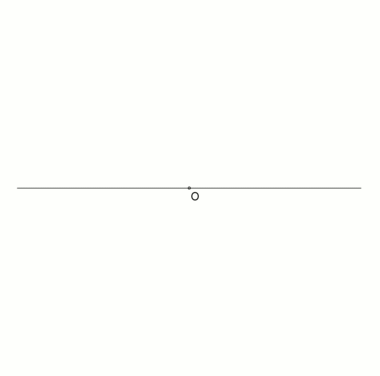An animation of a neusis construction of a heptagon with radius of circumcircle, based on Andrew M. Gleason, using angle trisection by means of the tomahawk:p. 186

A cubic equation with real coefficients can be solved geometrically with compass, straightedge, and an angle trisector if and only if it has three real roots.:Thm. 1

A regular polygon with n sides can be constructed with ruler, compass, and angle trisector if and only ifwhere r, s, k ≥ 0 and where the pi are distinct primes greater than 3 of the form:Thm. 2

## Generalization

For any nonzero integer N, an angle of measure 2πN radians can be divided into n equal parts with straightedge and compass if and only if n is either a power of 2 or is a power of 2 multiplied by the product of one or more distinct Fermat primes, none of which divides N. In the case of trisection (n = 3, which is a Fermat prime), this condition becomes the above-mentioned requirement that N not be divisible by 3.

## References

1. Dudley, Underwood (1994), The trisectors, Mathematical Association of America, ISBN 0-88385-514-3
2. Wantzel, P M L (1837). "Recherches sur les moyens de reconnaître si un problème de Géométrie peut se résoudre avec la règle et le compas." (PDF). Journal de Mathématiques Pures et Appliquées. 1. 2: 366–372. Retrieved 3 March 2014.
3. For the historical basis of Wantzel's proof in the earlier work of Ruffini and Abel, and its timing vis-a-vis Galois, see Smorynski, Craig (2007), History of Mathematics: A Supplement, Springer, p. 130, ISBN 9780387754802.
4. MacHale, Desmond. "Constructing integer angles", Mathematical Gazette 66, June 1982, 144–145.
5. McLean, K. Robin, "Trisecting angles with ruler and compasses", Mathematical Gazette 92, July 2008, 320–323. See also Feedback on this article in vol. 93, March 2009, p. 156.
6. Stewart, Ian (1989). Galois Theory. Chapman and Hall Mathematics. pp. g. 58. ISBN 0-412-34550-1.
7. Jim Loy (2003) . "Trisection of an Angle". Archived from the original on February 25, 2012. Retrieved 30 March 2012.
8. Yates, Robert C (1942). The Trisection Problem (PDF). The National Council of Teachers of Mathematics. pp. 39–42.
9. Trisection of an Angle Archived November 4, 2013, at the Wayback Machine.
10. Hutcheson, Thomas W. (May 2001). "Dividing Any Angle into Any Number of Equal Parts". Mathematics Teacher. 94 (5): 400–405.
11. Isaac, Rufus, "Two mathematical papers without words", Mathematics Magazine 48, 1975, p. 198. Reprinted in Mathematics Magazine 78, April 2005, p. 111.
12. Gleason, Andrew Mattei (March 1988). "Angle trisection, the heptagon, and the triskaidecagon" (PDF). The American Mathematical Monthly. 95 (3): 185–194. doi:10.2307/2323624. Archived from the original (PDF) on November 5, 2014.